# 1st PUC Computer Science Question Bank Chapter 3 Data Representation

## Karnataka 1st PUC Computer Science Question Bank Chapter 3 Data Representation

### 1st PUC Computer Science Data Representation One Mark Questions and Answers

Question 1.
Define number or numeral system.
It is a system of naming or representing numbers, as the decimal system or the binary system.

Question 2.
Give the classification of number systems.
Non-positional number system and Positional number system.

Question 3.
What is non-positional number system?
Anon-positional number system does not rely on the position of the number, but rather symbols to dictate the value of a number.

Question 4.
Give the drawback of non-positional number system.
Non-positional number system cannot be used to do arithmetic calculations effectively.

Question 5.
What is positional number system?
It is a system of writing numbers in which the position of a digit affects its value.

Question 6.
Mention any two positional number systems
Decimal number system and Binary number system.Question 7.
What do you mean by the base of the number system?
The radix or base is the number of unique digits, including zero that a positional numeral system uses to represent numbers.

Question 8.
Expand BIT.
Binary digit.

Question 9.
Define MSB.
The highest weight of the digit in a number is called Most Significant Bit.

Question 10.
Define LSB.
The least weight of the digit in a number is called Least Significant Bit.

Question 11.
What is the weight of the LSB in the octal number system?
The weight of the LSB of an octal number system is 0.

Question 12.
What is the weight of the MSB of the hexadecimal number system?
The weight of the MSB of the hexadecimal number system is 15.

Question 13.
What is the Decimal number system?
The positional number system that has 10 digits which are 0,1,2,3,4,5,6,7,8 and 9 is called decimal number system. Decimal is also called base 10 because it has 10 digits.

Question 14.
What is the binary number system?
A positional number system that has only 2 digits which are 0 and 1, is called a binary number system it is also called as base 2.

Question 15.
What is octal number system?
A positional number system that has 8 digits which are 0,1,2,3,4,5,6 and 7 is called octal number system. It is also called a base 8.

Question 16.
What is the hexadecimal number system?
A positional number system that has 16 digits which are 0,1,2,3,4,5,6,7,8,9, A, B, C, D, E, and F is called hexadecimal number system which is also called as base 16.

Question 17.
How are positive or negative signs of a number represented in the binary system?
Add an extra digit at the front of the binary number, to indicate whether the number is positive or negative. This digit is called a sign bit.

Question 18.
How is negative number represented in binary number system?
A negative number is represented in binary number system by adding an extra digit 1 at the front of binary number.Question 19.
Expand BCD.
Binary Coded Decimal.

Question 20.
Write the expansion of ASCII.
American Standard Code of Information Interchange.

Question 21.
Expand EBCDIC?
Extended Binary Coded Decimal Interchange Code.

Question 22.
What is one’s complement?
Representing a signed number with 1’s complement is done by changing all the bits that are 1 to 0 and all the bits that are
0 to 1.

Question 23.
What is two’s complement?
Representing a signed number with 2’s complement is done by adding 1 to the 1’s complement representation of the number.

### 1st PUC Computer Science Data Representation Two/Three Marks Questions and Answers

Question 1.
What is the different between non-positional number system and positional number system?
The non-positional number system consists of different symbols and each of these symbols represents a value irrespective of its position.
In positional number system, each digit gets its value by its position in the digits.

Question 2.
How is a number represented in positional number system? Give an example.
The decimal number 259 is represented as
2 × 10² + 5 × 101 + 9 × 100
= (2 × 100) + (5 × 10) +(9 × 1)
= 200 + 50 + 9 = 259.

Question 3.
Write the different types of positional number system.

• Decimal number system
• Binary number system.
• Octal number system.

Question 4.
A binary number system is used in computers. Why?
Computer systems use electronic circuits which exist in only one of two states i.e., on a state or off state. The binary number system is a method of representing numbers that count by using combinations of only two numerals: zero (0) and one (1).

Question 5.
What is the use of the hexadecimal number system?
The larger binary digits are not easier to remember and work with. But the hexadecimal system is much easier to work with compared to dealing with 16 0s and 1 s in the binary system.

Question 6.
What is 1’s complement? Give an example.
Representing a signed number with 1’s complement is done by changing all the bits that are 1 to 0 and all the bits that are
0 to 1. For example, 1’s complement of 1010100 is 0101011.Question 7.
What is two’s complement? Give an example.
Representing a signed number with 2’s complement is done by adding 1 to the 1’s complement representation of the number. For example, 2’s complement of 1010100 is
Step 1: 1’s complement of 1010100 is 0101011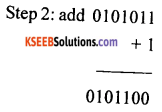Question 8.
What are computer codes? Give an example.
It is a set of symbols for representing characters. For example, most computers use ASCII codes to represent characters.

Question 9.
Why binary number code is used in computers?
The term “binary” means “two”. Thus, the binary number system is a system of numbers based on two possible digits – 0 and 1. Each binary digit, or “bit”, is a single 0 or 1, which directly corresponds to a single “switch” in a circuit. Binary codes are used in computers because they are used to represent information in the digital world.

Question 10.
What is the weighted code in the binary number system?
Weighted binary codes are those binary codes which obey the positional weight principle. Each position of the number represents a specific weight. Several systems of the codes are used to express the decimal digits 0 through 9. In these codes, each decimal digit is represented by a group of four bits.

Question 11.
What are non-weighted codes? Give an example.
In this type of binary code, the positional weights are not assigned. The examples of nonweighted codes are Excess-3 code and Gray code.

Question 12.
Write the binary equivalent of decimal 61.Question 13.
Write the binary equivalent of decimal
28(10) = 11100(2)25(10) = 01(2)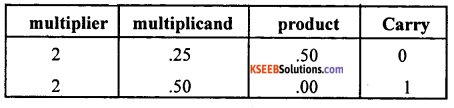Question 14.
Write the octal equivalent of decimal 65.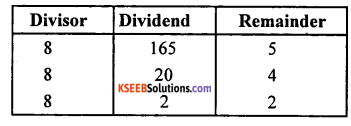Question 15.
Write the hexadecimal equivalent of decimal 345.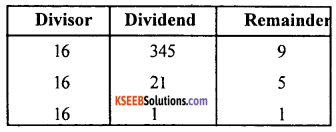Question 16.
Convert 101101(2) to decimal.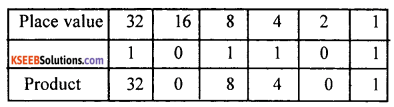32 + 8 + 4 +1 =45

Question 17.
Convert 101110(2) to octal.Question 18.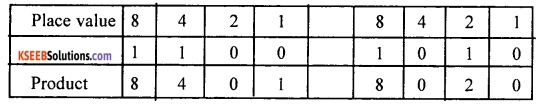8 + 4+1 = 13 in hexadecimal is D 8 + 2 = 10 in hexadecimal is A

Question 19.
Convert 3257(8) to decimal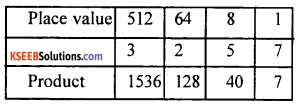1536 + 128 + 40 + 7 = 1711(10)

Question 20.
Convert 357(8) to binary.011 101 111(2)

Question 21.
Step 1 : 357(8) = 011 101 111(2)
Step 2: 11101111… group of 4 binary digits from LSB is 1110(2) 1111(2)8 + 4 + 2 = 14(10) = E(16) 8 + 4+ 2+ 1 = 15(10) =F(16)
= EF(16)

Question 22.
Convert FACE(16) to decimal.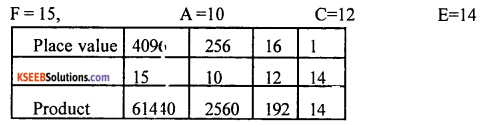61440 + 2560 + 192 + 14 = 64206(10)

Question 23.
Write the sum of 1100(2) and 101(2)
1100(2) + 101(2) = 10001(2)

Question 24.
Name the two types of ASCII codes.
ASCII 7 bit and ASCII 8 bit are the two types.

Question 25.
What is EBCDIC code?
EBCDIC (Extended Binary Coded Decimal Interchange Code is a binary code for alphabetic and numeric characters that IBM developed for its larger operating systems. In an EBCDIC file, each alphabetic or numeric character is represented by an 8-bit binary number. 256 possible characters (letters of the alphabet, numerals, and special characters) are defined.

### 1st PUC Computer Science Data Representation Five Marks Questions and Answers

Question 1.
Write a short note on the number of systems.
It is a system of naming or representing numbers, as the decimal system or the binary system. The number system is broadly classified into two types. Namely, positional and non-positional number systems.
1. Non-positional number system:
A non-positional number system does not rely on the position of the number, but rather symbols to dictate the value of a number. Roman number is a non-positional number system. Non-positional number system cannot be used to do arithmetic calculations effectively.

2. Positional number system:
It is a system of writing numbers in which the position of a digit affects its value. The Decimal number system and Binary number system are examples of a positional number system. The positional number system can be used to do arithmetic calculations effectively.Question 2.
What is the radix or base of a number system? Give the radix of different number systems.
The radix or base is the number of unique digits, including zero that a positional numeral
system uses to represent numbers.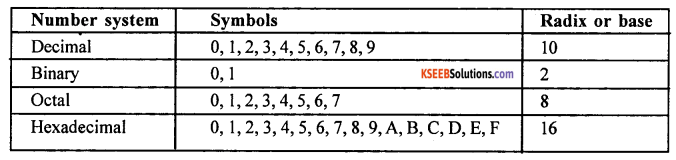Question 3.
Convert 2567(10) = (?)(2) = (? )(8) = ( ? )(16)
Binary equivalent of 2567(10) is 101000000111(2)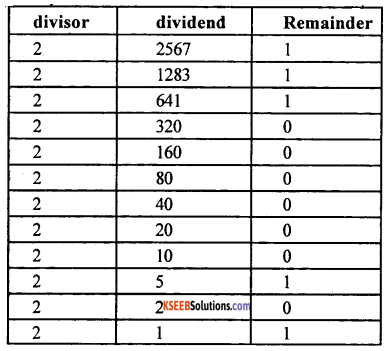Octal equivalent of 2567(10) is 5007(8)
2567(10) binary in broken into set of three bits is 101 000 000 111
5(8) 0(8) 0 (8) 7(8)
Hexadecimal equivalent of 2567(10) is A07(16)
2567(10) binary is divided into set of four bits is 1010 0000 0111
A(16) 0(16) 7(16)

Question 4.
Write a short note on binary arithmetic.
Binary arithmetic is essential part of all digital computers and many other digital system.
It is a key for binary subtraction, multiplication, division. The four rules of the binary addition are.
0 + 0 = 0
0 + 1 = 1
1 + 0 = 1
1 + 1 = 0 with a carry bit 1.

2. Binary Subtraction:
Subtraction and Borrow, these two words will be used very frequently for the binary subtraction.There are four rules of the binary substration. The four rules of the binary Subtraction are
0 – 0 = 0
0 – 1 = 0 with a borrow 1
1 – 0 = 1
1 – 1 = 0

Question 5.
Write a note on binary system complements.
Complements are used in the digital computers in order to simplify the subtraction operation and for logical manipulations. The two types of complements are 1’s complement and 2’s complement.
1. 1’s complement:
The 1 ’s complement of a number is found by changing all 1’s to 0’s and all 0’s to 1’s. This is called as taking complement or 1’s complement. For example, 10101 is 01010 in 1’s complement.

2. 2’s complement:
The 2’s complement of binary number is obtained by adding 1 to the Least Significant Bit (LSB) of 1 ’s complement of the number.
2’s complement = 1’s complement + 1
For example, 10101(2)
1’s complement is 01010 and
01010 + 1 = 01011 is 2’s complement.

Question 6.
Explain in brief binary subtraction using l’s complement.
Subtraction with One’s Complement:-
We can subtract the 5-bit binary numbers 01101(2) 13(10) and 01001(2) 9(10)by converting 01001(10) to its negative equivalent in 1’s complement and adding this value to 011012.
1. First, we need to convert 01001(2) to its negative equivalent in 1 ’s complement.2. To do this we change all the 1’s to 0’s and 0’s to 1’s. Notice that the most-significant digit is now 1 since the number is negative.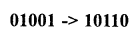3. Next, we add the negative value we computed to 011012. This gives us a result of 1000112.4. Notice that our addition caused an overflow bit. Whenever we have an overflow bit in 1’s complement, we add this bit to our sum to get the correct answer. This gives us a final answer of 001002 (or 410).Question 7.
Explain in brief binary subtraction using 2’s complement.
Subtraction with Two’s Complement:-
We can subtract the 5-bit binary numbers 011012 (1310) and 010012 (910) by converting 010012 to its negative equivalent in 2’s complement and adding this value to 011012.
1. First, we need to convert 010012 to its negative equivalent in 2’s complement.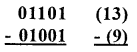2. To do this first we change all the 1s to 0s and vice versa.3. Then we add 1 to the number to obtain our negative equivalent. Notice that the most-significant digit is now 1 since the number is negative.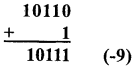4. Next, we add the negative value we computed to 011012. This gives us a result of 1001002. However, since we are only using 5-bit binary numbers, we discard the extra bit on the left side.5. This gives us a final answer of 001002 (or 4).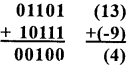Question 8.
Write a note on BCD and Excess-3 code.
1. Binary Coded Decimal (BCD) code:
In this code, each decimal digit is represented by a 4-bit binary number. BCD is a way to express each of the decimal digits with a binary code. In the BCD, with four bits we can represent sixteen numbers (0000 to 1111). But in BCD code only the first ten of these are used (0000 to 1001). The remaining six code combinations i.e. 1010 to 1111 are invalid in BCD. Justify

2. Excess-3 code:
The Excess-3 code is also called an XS-3 code. It is a non-weighted code used to express decimal numbers. The Excess-3 code numbers derived from the 8421 BCD code numbers adding (0011)2 or (3)10 to each codeword in 8421. The excess-3 codes are obtained as follows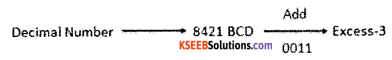Question 9.
Write a note on ASCII and EBCDIC code.